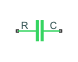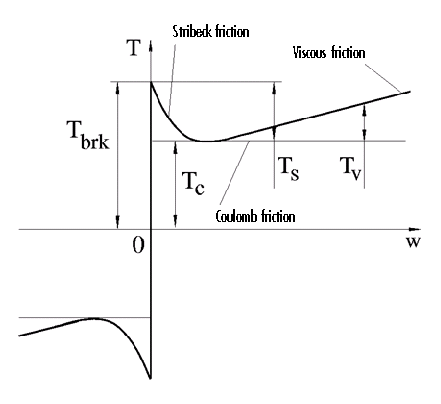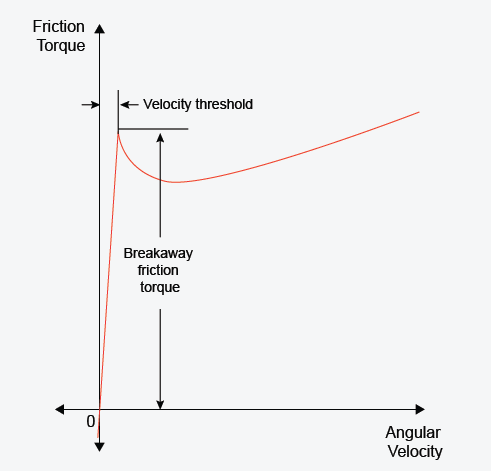# Rotational Friction

Friction in contact between rotating bodies

•Libraries:
Simscape / Foundation Library / Mechanical / Rotational Elements

## Description

The Rotational Friction block represents friction in contact between rotating bodies. The friction torque is simulated as a function of relative velocity and is assumed to be the sum of Stribeck, Coulomb, and viscous components, as shown in the following figure.The Stribeck friction, TS, is the negatively sloped characteristics taking place at low velocities . The Coulomb friction, TC, results in a constant torque at any velocity. The viscous friction, TV, opposes motion with the torque directly proportional to the relative velocity. The sum of the Coulomb and Stribeck frictions at the vicinity of zero velocity is often referred to as the breakaway friction, Tbrk. The friction is approximated with the following equations:

`$T=\sqrt{2e}\left({T}_{brk}-{T}_{C}\right)\cdot \mathrm{exp}\left(-{\left(\frac{\omega }{{\omega }_{St}}\right)}^{2}\right)\cdot \frac{\omega }{{\omega }_{St}}+{T}_{C}\cdot \mathrm{tanh}\left(\frac{\omega }{{\omega }_{Coul}}\right)+f\omega$`
`${\omega }_{St}={\omega }_{brk}\sqrt{2}$`
`${\omega }_{Coul}={\omega }_{brk}/10$`
`$\omega ={\omega }_{R}-{\omega }_{C}$`

where

• T is friction torque.

• TC is Coulomb friction torque.

• Tbrk is breakaway friction torque.

• ωbrk is breakaway friction velocity.

• ωSt is Stribeck velocity threshold.

• ωCoul is Coulomb velocity threshold.

• ωR and ωC are absolute angular velocities of ports R and C, respectively.

• ω is relative velocity.

• f is viscous friction coefficient.

The exponential function used in the Stribeck portion of the force equation is continuous and decays at velocity magnitudes greater than the breakaway friction velocity.

The hyperbolic tangent function used in the Coulomb portion of the force equation ensures that the equation is smooth and continuous through ω = 0, but quickly reaches its full value at nonzero velocities.

The block positive direction is from port R to port C. This means that if the port R velocity is greater than that of port C, the block transmits torque from R to C.

### Variables

To set the priority and initial target values for the block variables prior to simulation, use the Initial Targets section in the block dialog box or Property Inspector. For more information, see Set Priority and Initial Target for Block Variables.

Nominal values provide a way to specify the expected magnitude of a variable in a model. Using system scaling based on nominal values increases the simulation robustness. Nominal values can come from different sources, one of which is the Nominal Values section in the block dialog box or Property Inspector. For more information, see Modify Nominal Values for a Block Variable.

## Ports

### Conserving

expand all

Mechanical rotational conserving port associated with the rod, that is, the moving body.

Mechanical rotational conserving port associated with the case, that is, the stationary body.

## Parameters

expand all

The breakaway friction torque, which is the sum of the Coulomb and the static frictions. It must be greater than or equal to the Coulomb friction torque value.

The angular velocity at which the Stribeck friction is at its peak. At this point, the sum of the Stribeck and Coulomb friction is the Breakaway friction torque. This parameter specifies the velocity threshold, which affects the tradeoff between the simulation accuracy and speed.The Coulomb friction torque, which is the friction that opposes rotation with a constant torque at any velocity.

Proportionality coefficient between the friction torque and the relative angular velocity. The parameter value must be greater than or equal to zero.

 Armstrong, B. and C.C. de Wit, Friction Modeling and Compensation, The Control Handbook, CRC Press, 1995.